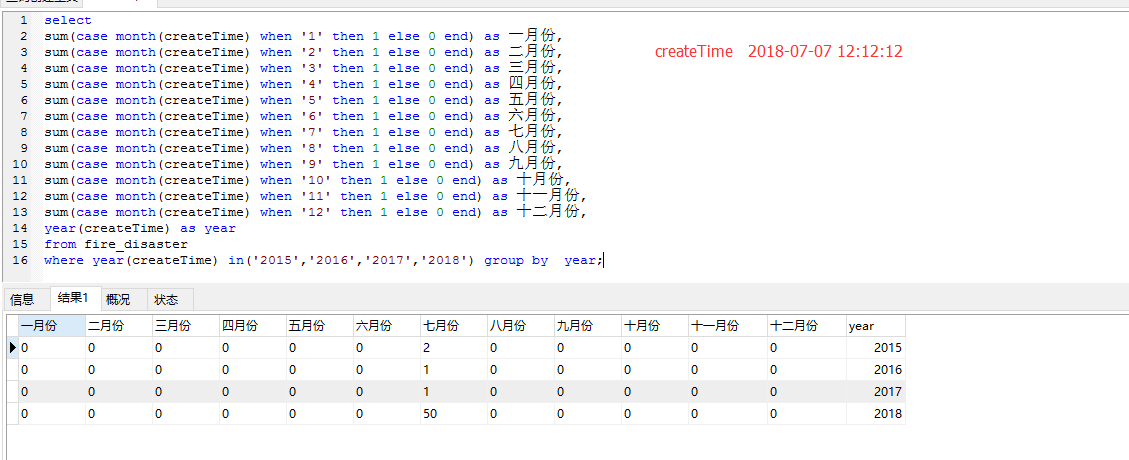# mysql统计一年12月的数据``````select
sum(case month(createTime) when '1' then 1 else 0 end) as 一月份,
sum(case month(createTime) when '2' then 1 else 0 end) as 二月份,
sum(case month(createTime) when '3' then 1 else 0 end) as 三月份,
sum(case month(createTime) when '4' then 1 else 0 end) as 四月份,
sum(case month(createTime) when '5' then 1 else 0 end) as 五月份,
sum(case month(createTime) when '6' then 1 else 0 end) as 六月份,
sum(case month(createTime) when '7' then 1 else 0 end) as 七月份,
sum(case month(createTime) when '8' then 1 else 0 end) as 八月份,
sum(case month(createTime) when '9' then 1 else 0 end) as 九月份,
sum(case month(createTime) when '10' then 1 else 0 end) as 十月份,
sum(case month(createTime) when '11' then 1 else 0 end) as 十一月份,
sum(case month(createTime) when '12' then 1 else 0 end) as 十二月份,
year(createTime) as year
from fire_disaster
where year(createTime) in('2015','2016','2017','2018') group by  year;
``````

TAG标签：
Ctrl+D 将本页面保存为书签，全面了解最新资讯，方便快捷。